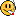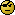## Impact of minimum stage 2 sample size on the TIE: example [Two-Stage / GS Designs]

Hi ElMaestro,

» » Simulating for power (at 0.95):
1. No lower limit of n2
» » library(Power2Stage)
» » power.2stage(method="B", alpha=rep(0.0294, 2), CV=0.2,
» »              n1=12, GMR=0.95, targetpower=0.8, min.n2=0)

» Should this not be min.n2=2 ?

Nope. This is the original Potvin ‘Method B’. There is no minimum n2 in the paper, right? However, the functions in Power2Stage are constructed in such a way that (in cross-over TSDs) any estimated sample size has to be an even number. If one states min.n2=1 it will automatically rounded up to 2. Same goes with sampleN.TOST(). Hence, to state min.n2=2 is a waste of time (see also the footnote to this post).

» Do you think you have it in your heart to explain in slow motion to a dimwit like me who read your posts quite a few times which point you are trying to prove or investigating?

I’ll try. Without a minimum n2 what would happen in a study which – following the conditions of the framework – could proceed to the second stage? n2 could be any even number. Say we had n1 24 and estimate the total sample size N (for stage 1 CV, assumed GMR and target power) with 30. Hence, n2 6.
If we mandate n2 = max(n2 = 1.5n1, Nn1) we have to perform the second stage in 36 instead of 6. In the pooled analysis we will have 60 subjects instead of 30. Much higher power (nice for wealthy sponsors) but not so nice if we look at the TIE. Since the final size is twice as large, the chance to pass BE (at 1.25) will be larger as well. Even if we keep everything equal the DFs come into play. Therefore, the ‘original’ adjusted α might not sufficiently control the TIE – and we would need a lower one.
That’s pure reasoning (wetware).» Otherwise I am afraid I will need to question you next time we meet f2f. And that might not be in the distant futureReally? Great!

Dif-tor heh smusma 🖖
Helmut SchützThe quality of responses received is directly proportional to the quality of the question asked. 🚮
Science QuotesIng. Helmut Schütz